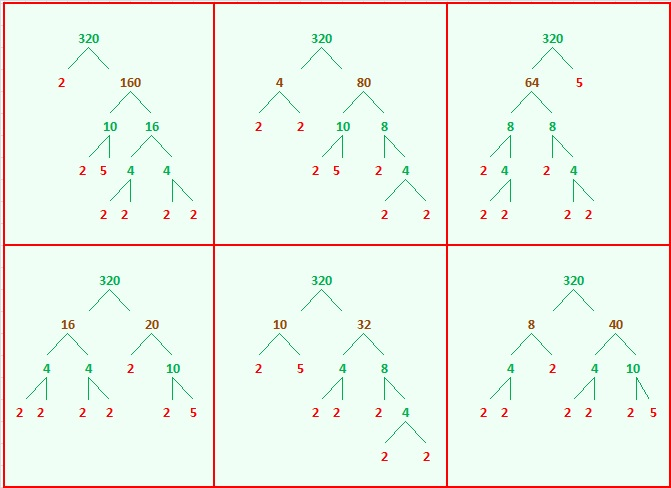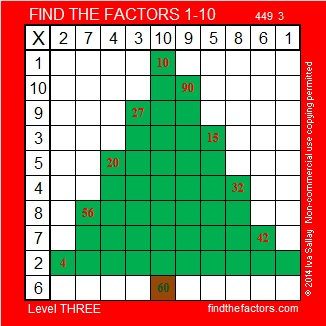# 320 Christmas Factor Trees

• 320 is a composite number.
• Prime factorization: 320 = 2 x 2 x 2 x 2 x 2 x 2 x 5, which can be written 320 = 2⁶ x 5
• The exponents in the prime factorization are 6, and 1. Adding one to each and multiplying we get (6 + 1)(1 + 1) = 7 x 2 = 14. Therefore 320 has exactly 14 factors.
• Factors of 320: 1, 2, 4, 5, 8, 10, 16, 20, 32, 40, 64, 80, 160, 320
• Factor pairs: 320 = 1 x 320, 2 x 160, 4 x 80, 5 x 64, 8 x 40, 10 x 32, or 16 x 20
• Taking the factor pair with the largest square number factor, we get √320 = (√5)(√64) = 8√5 ≈ 17.889In these factor trees for 320, we can also see factor trees for 4, 8, 10, 16, 20, 32, 40, 64, 80, and 160. The tops of each of those factor trees are in brown. No matter which factor pairs we use to build the tree, we always get the same prime factors which are indicated in red.Now try finding the factors in this Christmas tree puzzle that make it work like a multiplication table:Print the puzzles or type the factors on this excel file: 10 Factors 2014-12-08

A Logical Approach to FIND THE FACTORS: Find the column or row with two clues and find their common factor. Write the corresponding factors in the factor column (1st column) and factor row (top row).  Because this is a level three puzzle, you have now written a factor at the top of the factor column. Continue to work from the top of the factor column to the bottom, finding factors and filling in the factor column and the factor row one cell at a time as you go.## One thought on “320 Christmas Factor Trees”

1.kate1975

Reblogged this on Kate Is Rising and commented:
I liked this post a lot and wanted to share it. Math Christmas trees! Excellent.

This site uses Akismet to reduce spam. Learn how your comment data is processed.